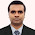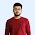## Java Array : Programming Exercise for Practice

The java array is one of the most important topics for programming interviews. It doesn't matter if you are going to Google, Microsoft, Amazon or even service based companies like IBM, Accenture, TCS, or Infosys, if you are going to interview for the developer position, you must prepare array very well. In fact, it is the second most topic after String and if you look closely, String coding problems can be solved by treating them like character array.

An array is a one of the data structure in Java, that can store a fixed-size sequential collection of elements of the same data type. It is also known as static data structure because size of an array must be specified at the time of its declaration. An array gets memory in heap area and Index of array starts from zero to size-1.

For many large applications, there may arise some situations that need a single name to store multiple values. To process such large amount of data, programmers need powerful data types that would facilitate the efficient contiguous bulk amount of storage facility, accessing and dealing with such data items. So, Java array is used.

>> Why you should learn Linux | programmer preferred & Best OS.

### List Of Java Array Programming Problems for Practice

1. Write a Java program to read and print elements of array. - using recursion.
2. Write a Java program to print all negative elements in an array.
3. Write a Java program to find sum of all array elements. - using recursion.
4. Write a Java program to calculate the average value of array elements.
5. Write a Java program to test if an array contains a specific value.
6. Write a Java program to find the index of an array element.
7. Write a Java program to find maximum and minimum element in an array.
8. Write a Java program to find second largest element in an array.
9. Write a Java program to find the second smallest element in an array.
10. Write a program to count total number of even and odd elements in an array.

11. Write a program to count total number of negative elements in an array.
12. Write a program to copy all elements from an array to another array.
13. Write a program to insert an element in an array.
14. Write a program to delete an element from an array at specified position.
15. Write a Java program to find the duplicate values of an array of integer values.
16. Write a program to print all unique elements in the array.
17. Write a program to count total number of duplicate elements in an array.
18. Write a program to delete all duplicate elements from an array.
19. Write a program to merge two array to third array.
20. Write a Java program to reverse an array of integer values.
21. Write a program to put even and odd elements of array in two separate array.
22. Write a program to search an element in an array.
23. Write a program to sort array elements in ascending or descending order.
24. Write a program to sort even and odd elements of array separately.
25. Write a program to left rotate an array.
26. Write a program to right rotate an array.
27. Write a program to find the common elements between two arrays (string values).
28. Write a program to find the common elements between two arrays of integers.
29. Write a Java program to print the pattern-
* * * * * * *
* * * * * * *
* * * * * * *
* * * * * * *
30. Write a Java program to print the pattern-
*
* *
* * *
* * * *
* * * * *
31. Write a Java program to print the pattern-
* * * * *
* * * *
* * *
* *
*
33. Write a Java program to print the pattern-
*
*   *
*   *   *
*   *   *   *
*   *   *   *   *

### List of matrix programming exercises :

34. Write a Java program to add two matrices.
35. Write a Java program to subtract two matrices.
36. Write a Java program to perform Scalar matrix multiplication.
37. Write a Java program to multiply two matrices.
38. Write a Java program to check whether two matrices are equal or not.
39. Write a Java program to find sum of main diagonal elements of a matrix.
40. Write a Java program to find sum of minor diagonal elements of a matrix.
41. Write a Java program to find sum of each row and column of a matrix.
42. Write a Java program to interchange diagonals of a matrix.
43. Write a Java program to find upper triangular matrix.
44. Write a Java program to find lower triangular matrix.
45. Write a Java program to find sum of upper triangular matrix.
46. Write a Java program to find sum of lower triangular matrix.
47. Write a Java program to find transpose of a matrix.
48. Write a Java program to find determinant of a matrix.
49. Write a Java program to check Identity matrix.
50. Write a Java
51. Write a Java program to convert an array to ArrayList.
52. Write a Java program to convert an ArrayList to an array.
53. Write a Java program to find all pairs of elements in an array whose sum is equal to a specified number.
54. Write a Java program to test the equality of two arrays.
55. Write a Java program to find a missing number from 1-9 in an array.
56. Write a Java program to move all 0's to the end of an array. Maintain the relative order of the other (non-zero) array elements.
57.Write a Java program to get the difference between the largest and smallest values in an array of integers.
58. Write a program to check if an array of integers contains two specified elements 65 and 77.
59. Write a Java program to check if the sum of all the 10's in the array is exactly 30. Return false if the condition does not satisfy, otherwise true.

60. Write a Java program to remove the duplicate elements of a given array and return the new length of the array.
Sample array: [20, 20, 30, 40, 50, 50, 50]
After removing the duplicate elements the program should return 4 as the new length of the array

61. Write a Java program to find the length of the longest consecutive elements sequence from a given unsorted array of integers.
Sample array: [49, 1, 3, 200, 2, 4, 70, 5]
The longest consecutive elements sequence is [1, 2, 3, 4, 5], therefore the program will return its length 5.

62. Write a Java program to find the sum of the two elements of a given array which is equal to a given integer.
Sample array: [1,2,4,5,6]
Target value: 6.

63. Write a Java program to find all the unique triplets such that sum of all the three elements [x, y, z (x ≤ y ≤ z)] equal to a specified number.
Sample array: [1, -2, 0, 5, -1, -4]
Target value: 2.

>> 10 Interesting Facts About Google You Should Be Know.

Thanks for reading this article "java array problems for practice" so far. If you like this article then please share with your friends and colleagues. If you have any question or doubt then please let us know and I'll try to find an answer for you.

Incoming Searches : java array questions, array practice question, array problems for practice, programming exercise for practice
Java Array : Programming Exercise for Practice [60+ Problems]Reviewed by Ashutosh on 10:22:00 PM Rating: 5

1.Mridha IT
Thank you, I’ve just been searching for information about this topic for a while and yours is the greatest I’ve discovered till now.

2.Mridha IT
Thank you, I’ve just been searching for information about this topic for a while and yours is the greatest I’ve discovered till now.

1.Thank you Shameen!!

3.Those are good question, and thanks for the share

how can i get the solutions?